The OpenFOAM Foundation
icoPolynomial< Specie, PolySize > Class Template Reference

Incompressible, polynomial form of equation of state, using a polynomial function for density. More...

Inheritance diagram for icoPolynomial< Specie, PolySize >: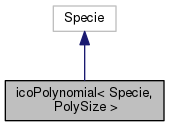[legend]
Collaboration diagram for icoPolynomial< Specie, PolySize >:[legend]

## Public Member Functions

icoPolynomial (const Specie &sp, const Polynomial< PolySize > &rhoPoly)
Construct from components. More...

icoPolynomial (Istream &)
Construct from Istream. More...

icoPolynomial (const dictionary &dict)
Construct from dictionary. More...

icoPolynomial (const icoPolynomial &)
Construct as copy. More...

icoPolynomial (const word &name, const icoPolynomial &)
Construct as named copy. More...

autoPtr< icoPolynomialclone () const
Construct and return a clone. More...

scalar rho (scalar p, scalar T) const
Return density [kg/m^3]. More...

scalar h (const scalar p, const scalar T) const
Return enthalpy departure [J/kmol]. More...

scalar cp (scalar p, scalar T) const
Return cp departure [J/(kmol K]. More...

scalar s (const scalar p, const scalar T) const
Return entropy [J/(kmol K)]. More...

scalar psi (scalar p, scalar T) const
Return compressibility rho/p [s^2/m^2]. More...

scalar Z (scalar p, scalar T) const
Return compression factor []. More...

scalar cpMcv (scalar p, scalar T) const
Return (cp - cv) [J/(kmol K]. More...

void write (Ostream &os) const
Write to Ostream. More...

void operator= (const icoPolynomial &)

void operator+= (const icoPolynomial &)

void operator-= (const icoPolynomial &)

void operator*= (const scalar)

## Static Public Member Functions

static autoPtr< icoPolynomialNew (Istream &is)

static autoPtr< icoPolynomialNew (const dictionary &dict)

static word typeName ()
Return the instantiated type name. More...

## Static Public Attributes

static const bool incompressible = true
Is the equation of state is incompressible i.e. rho != f(p) More...

static const bool isochoric = false
Is the equation of state is isochoric i.e. rho = const. More...

## Friends

icoPolynomial operator+ (const icoPolynomial &, const icoPolynomial &)

icoPolynomial operator- (const icoPolynomial &, const icoPolynomial &)

icoPolynomial operator* (const scalar s, const icoPolynomial &)

icoPolynomial operator== (const icoPolynomial &, const icoPolynomial &)

Ostreamoperator (Ostream &, const icoPolynomial &)

## Detailed Description

### template<class Specie, int PolySize = 8> class Foam::icoPolynomial< Specie, PolySize >

Incompressible, polynomial form of equation of state, using a polynomial function for density.

Source files

Definition at line 50 of file icoPolynomial.H.

## Constructor & Destructor Documentation

 icoPolynomial ( const Specie & sp, const Polynomial< PolySize > & rhoPoly )
inline

Construct from components.

Definition at line 32 of file icoPolynomialI.H.

References Foam::name().

Here is the call graph for this function: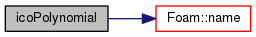icoPolynomial ( Istream & is )

Construct from Istream.

Definition at line 37 of file icoPolynomial.C.

 icoPolynomial ( const dictionary & dict )

Construct from dictionary.

Definition at line 47 of file icoPolynomial.C.

 icoPolynomial ( const icoPolynomial< Specie, PolySize > & )
inline

Construct as copy.

 icoPolynomial ( const word & name, const icoPolynomial< Specie, PolySize > & )
inline

Construct as named copy.

## Member Function Documentation

 Foam::autoPtr< Foam::icoPolynomial< Specie, PolySize > > clone ( ) const
inline

Construct and return a clone.

Definition at line 69 of file icoPolynomialI.H.

 Foam::autoPtr< Foam::icoPolynomial< Specie, PolySize > > New ( Istream & is )
inlinestatic

Definition at line 80 of file icoPolynomialI.H.

 Foam::autoPtr< Foam::icoPolynomial< Specie, PolySize > > New ( const dictionary & dict )
inlinestatic

Definition at line 91 of file icoPolynomialI.H.

References dict, and icoPolynomial< Specie, PolySize >::rho().

Here is the call graph for this function: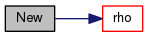static word typeName ( )
inlinestatic

Return the instantiated type name.

Definition at line 140 of file icoPolynomial.H.

 Foam::scalar rho ( scalar p, scalar T ) const
inline

Return density [kg/m^3].

Definition at line 104 of file icoPolynomialI.H.

References icoPolynomial< Specie, PolySize >::h().

Referenced by icoPolynomial< Specie, PolySize >::New().

Here is the call graph for this function: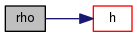Here is the caller graph for this function: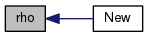Foam::scalar h ( const scalar p, const scalar T ) const
inline

Return enthalpy departure [J/kmol].

Definition at line 115 of file icoPolynomialI.H.

References icoPolynomial< Specie, PolySize >::cp().

Referenced by icoPolynomial< Specie, PolySize >::rho().

Here is the call graph for this function: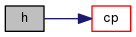Here is the caller graph for this function: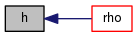Foam::scalar cp ( scalar p, scalar T ) const
inline

Return cp departure [J/(kmol K].

Definition at line 126 of file icoPolynomialI.H.

References icoPolynomial< Specie, PolySize >::s().

Referenced by icoPolynomial< Specie, PolySize >::h().

Here is the call graph for this function: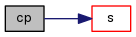Here is the caller graph for this function: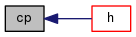Foam::scalar s ( const scalar p, const scalar T ) const
inline

Return entropy [J/(kmol K)].

Definition at line 137 of file icoPolynomialI.H.

References icoPolynomial< Specie, PolySize >::psi().

Referenced by icoPolynomial< Specie, PolySize >::cp().

Here is the call graph for this function: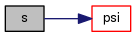Here is the caller graph for this function: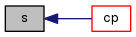Foam::scalar psi ( scalar p, scalar T ) const
inline

Return compressibility rho/p [s^2/m^2].

Definition at line 148 of file icoPolynomialI.H.

References icoPolynomial< Specie, PolySize >::Z().

Referenced by icoPolynomial< Specie, PolySize >::s().

Here is the call graph for this function: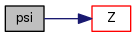Here is the caller graph for this function: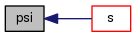Foam::scalar Z ( scalar p, scalar T ) const
inline

Return compression factor [].

Definition at line 159 of file icoPolynomialI.H.

References icoPolynomial< Specie, PolySize >::cpMcv().

Referenced by icoPolynomial< Specie, PolySize >::psi().

Here is the call graph for this function: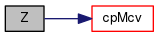Here is the caller graph for this function: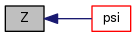Foam::scalar cpMcv ( scalar p, scalar T ) const
inline

Return (cp - cv) [J/(kmol K].

Definition at line 170 of file icoPolynomialI.H.

References Foam::sqr().

Referenced by icoPolynomial< Specie, PolySize >::Z().

Here is the call graph for this function: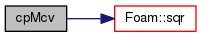Here is the caller graph for this function: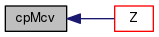void write ( Ostream & os ) const

Write to Ostream.

Definition at line 65 of file icoPolynomial.C.

References dictionary::add(), dict, dictionaryName::dictName(), Foam::indent(), Foam::name(), and write().

Here is the call graph for this function: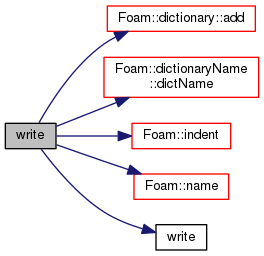void operator= ( const icoPolynomial< Specie, PolySize > & )
inline

Definition at line 183 of file icoPolynomialI.H.

 void operator+= ( const icoPolynomial< Specie, PolySize > & )
inline

Definition at line 195 of file icoPolynomialI.H.

 void operator-= ( const icoPolynomial< Specie, PolySize > & )
inline

Definition at line 212 of file icoPolynomialI.H.

 void operator*= ( const scalar s )
inline

Definition at line 228 of file icoPolynomialI.H.

References s().

Here is the call graph for this function: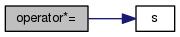## Friends And Related Function Documentation

 icoPolynomial operator+ ( const icoPolynomial< Specie, PolySize > & , const icoPolynomial< Specie, PolySize > & )
friend
 icoPolynomial operator- ( const icoPolynomial< Specie, PolySize > & , const icoPolynomial< Specie, PolySize > & )
friend
 icoPolynomial operator* ( const scalar s, const icoPolynomial< Specie, PolySize > & )
friend
 icoPolynomial operator== ( const icoPolynomial< Specie, PolySize > & , const icoPolynomial< Specie, PolySize > & )
friend
 Ostream& operator ( Ostream & , const icoPolynomial< Specie, PolySize > & )
friend

## Member Data Documentation

 const bool incompressible = true
static

Is the equation of state is incompressible i.e. rho != f(p)

Definition at line 149 of file icoPolynomial.H.

 const bool isochoric = false
static

Is the equation of state is isochoric i.e. rho = const.

Definition at line 152 of file icoPolynomial.H.

The documentation for this class was generated from the following files:
• src/thermophysicalModels/specie/equationOfState/icoPolynomial/icoPolynomial.H
• src/thermophysicalModels/specie/equationOfState/icoPolynomial/icoPolynomial.C
• src/thermophysicalModels/specie/equationOfState/icoPolynomial/icoPolynomialI.H